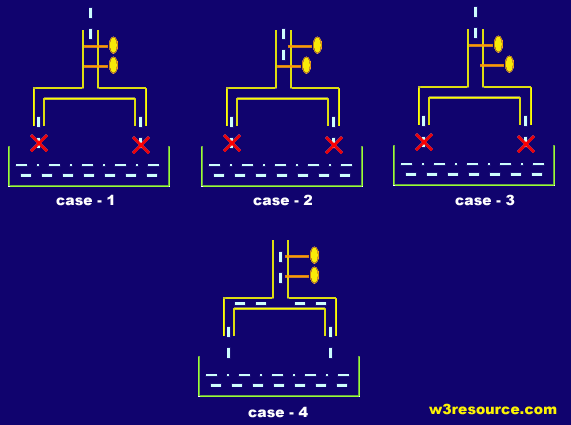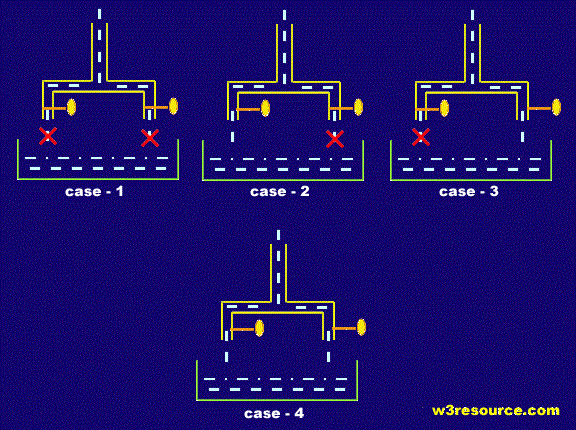# PHP : Logical Operators

## Description

The standard logical operators and, or, not, and xor are supported by PHP. Logical operators first convert their operands to boolean values and then perform the respective comparison.

Here is the list of logical operators :

Operator Name Example Result
&& and \$x && \$y is true if both \$x and \$y are true.
|| or \$x || \$y is true if either \$x or \$y is true.
xor xor \$x xor \$y is true if either \$x or \$y are true, but not both.
! not !\$x is true if \$x is not true.
and and \$x and \$y is true if both \$x and \$y are true.
or or \$x or \$y is true if either \$x or \$y is true.

PHP logical && operatorThis above pictorial helps you to understand the concept of LOGICAL AND operation with an analogy of taps and water.

In case-1 of the picture, both of the taps are closed, so the water is not flowing down. Which explains that if both of conditions are FALSE or 0, the return is FALSE or 0.

In case-2 of the picture, one of the taps are closed, even then, the water is not flowing down. Which explains that even if any of conditions are FALSE or 0, the return is FALSE or 0.

case-3 of the picture resembles CASE -2.

In case-4 of the picture, both of the taps are open, so the water is flowing down. Which explains that if both of conditions are TRUE or 1, the return is TRUE or 1.

So we can conclude that if and only if, both of the conditions are TRUE or 1, LOGICAL AND operations returns TRUE or 1.

PHP logical || operatorThe above pictorial helps you to understand the concept of LOGICAL OR operation with an analogy of taps and water.

In case-1 of the picture, both of the taps are closed, so the water is not flowing down. Which explains that if both of conditions are FALSE or 0, the return is FALSE or 0.

In case-2 of the picture, one of the taps are closed, and we can see that the water is flowing down. Which explains that if any of conditions are TRUE or 1, the return is TRUE or 1.

case-3 of the picture resembles CASE -2.

In case-4 of the picture, both of the taps are open, so the water is flowing down. Which explains that if both of conditions are TRUE or 1, the return is TRUE or 1.

So we can conclude that in LOGICAL OR operation if any of the conditions are true, the output is TRUE or 1.

Example :

``````<?php
\$a = true && false;
var_dump(\$a);
\$b = false && true;
var_dump(\$b);
\$c = true && true;
var_dump(\$c);
\$d = false && false;
var_dump(\$d);
\$a = true || false;
var_dump(\$a);
\$b = false || true;
var_dump(\$b);
\$c = true || true;
var_dump(\$c);
\$d = false || false;
var_dump(\$d);
?>``````

Output :

```bool(false)
bool(false)
bool(true)
bool(false)
bool(true)
bool(true)
bool(true)
bool(false)```

View the example in the browser

Previous: Comparison Operators
Next: Assignment Operators

﻿

## PHP: Tips of the Day

Variables can be incremented or decremented by 1 with ++ or --, respectively. They can either precede or succeed variables and slightly vary semantically, as shown below.

Example:

```\$i = 1;
echo \$i; // Prints 1
// Pre-increment operator increments \$i by one, then returns \$i
echo ++\$i; // Prints 2
// Pre-decrement operator decrements \$i by one, then returns \$i
echo --\$i; // Prints 1
// Post-increment operator returns \$i, then increments \$i by one
echo \$i++; // Prints 1 (but \$i value is now 2)
// Post-decrement operator returns \$i, then decrements \$i by one
echo \$i--; // Prints 2 (but \$i value is now 1)
```